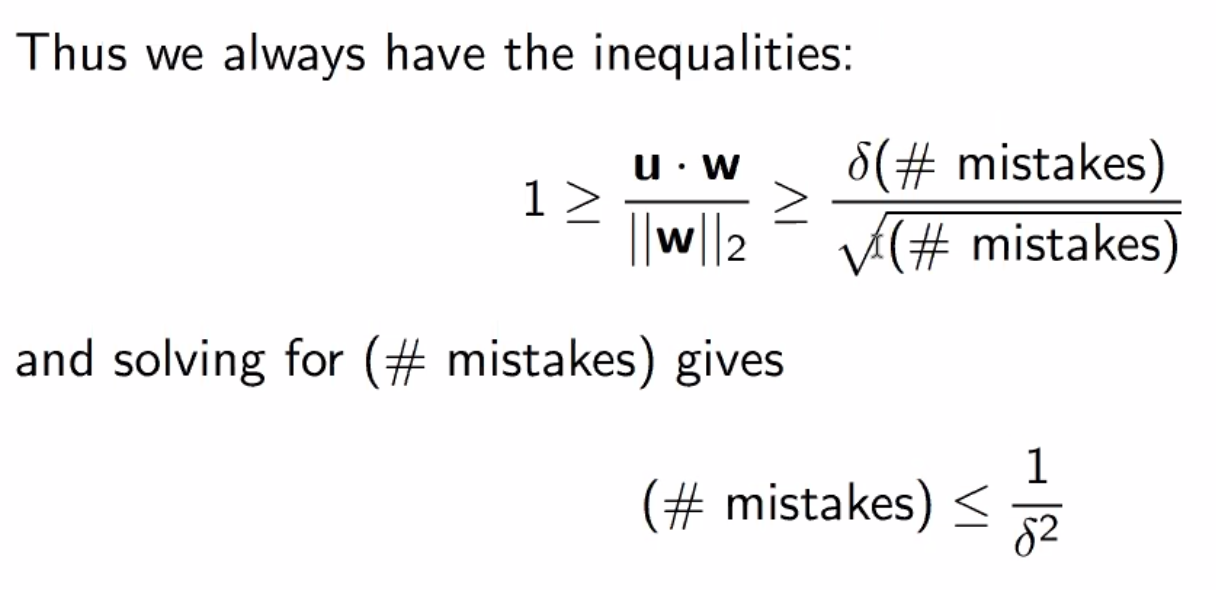# Perceptron¶

Perceptron is a linear, online classification model

Given a training set of pairs, it learns a linear decision boundary hyperplane - we assume labels are binary for now

It’s inspired by neurons: activation is a function of its inputs and weights. For example, the weighted sum activation:

$activation = \sum_{i=1}^D w_ix_i$

Then, prediction can be something like a > 0 ? 1 : -1.

Additionally, we can add a bias term to account for a non-zero intercept:

$a = [\sum_{i=1}^D w_ix_i] + b$

## Linear Boundary¶

• a D-1 dimensional hyperplane separates a D dimensional space into two half-spaces: positive and negative
• this linear boundary has the form $$\mathbf{w} \cdot \mathbf{x} = 0$$
• defined by w: the unit vector (often normalized) normal to any vector on the hyperplane
• $$\text{proj}_w x$$ is how far away x is from the decision boundary
• when w is normalized to a unit vector, $$\mathbf{w} \cdot \mathbf{x} = \text{proj}_w x$$.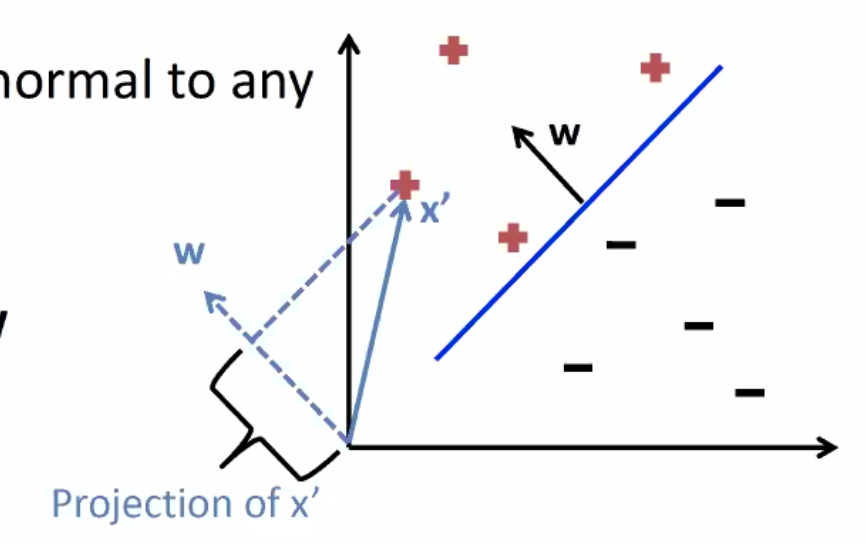With Bias

• When a bias is added, the linear boundary becomes $$\mathbf{w} \cdot \mathbf{x} + b = 0$$
• this can be converted to the more general form $$\mathbf{w} \cdot \mathbf{x} = 0$$ by adding b to w and an always-1 feature to x

## Prediction¶

Pretty simple:

def prediction(w, x, b):
return sign( w @ x + b )


## Training¶

This is an error-driven model:

1. initialize model to some weights and biases
2. for each instance in training set:
1. use current w and b to predict a label $$\hat{y}$$
2. if $$\hat{y} = y$$ do nothing
3. otherwise update w and b to do better
3. goto 2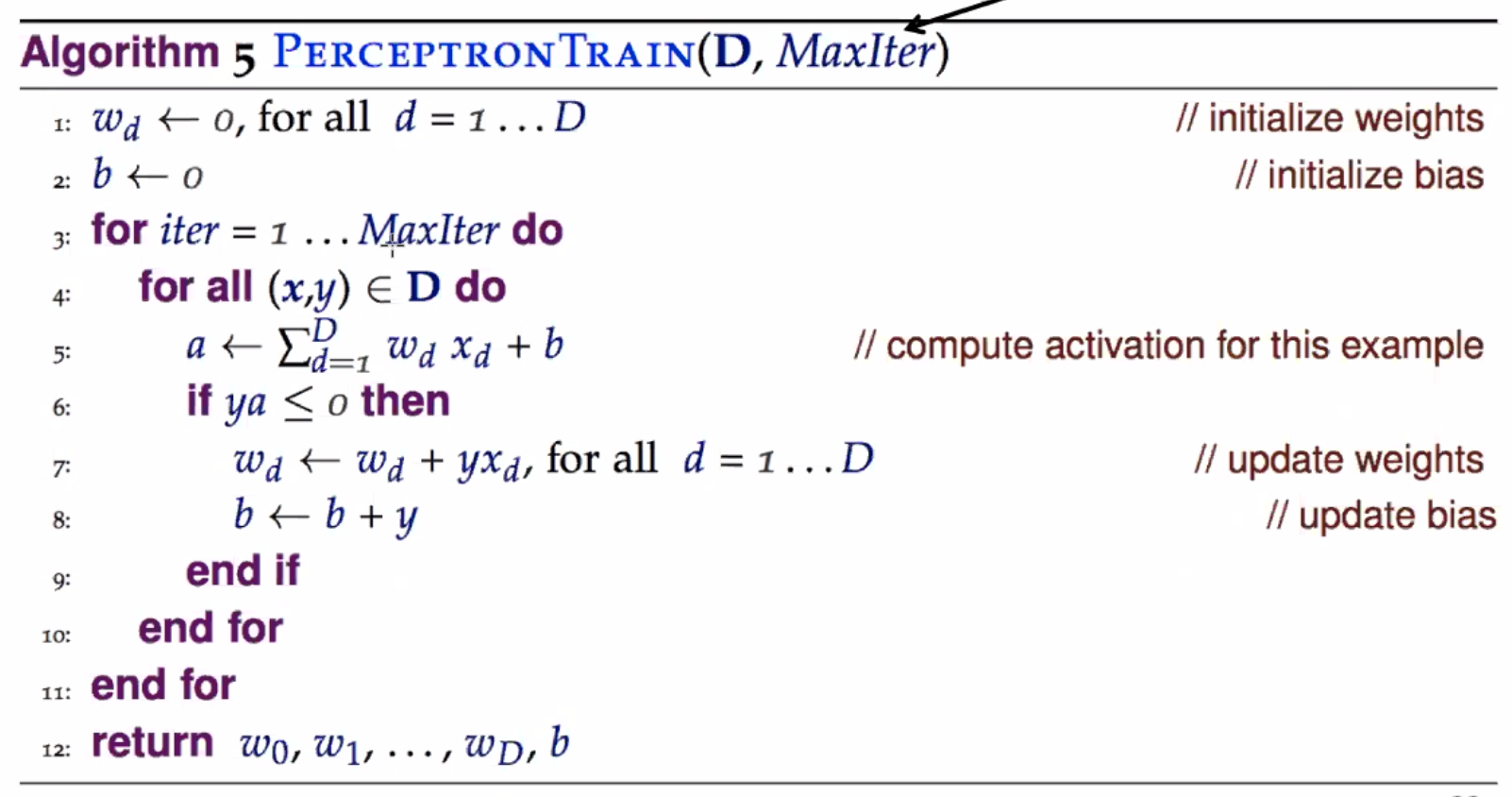Update in a little simpler notation:

$\begin{split}\mathbf{w} & = \mathbf{w} + y \mathbf{x} \\ b & = b + y\end{split}$

So what does it do? Let’s look at the new activation after an update where a positive was incorrectly predicted as a negative label: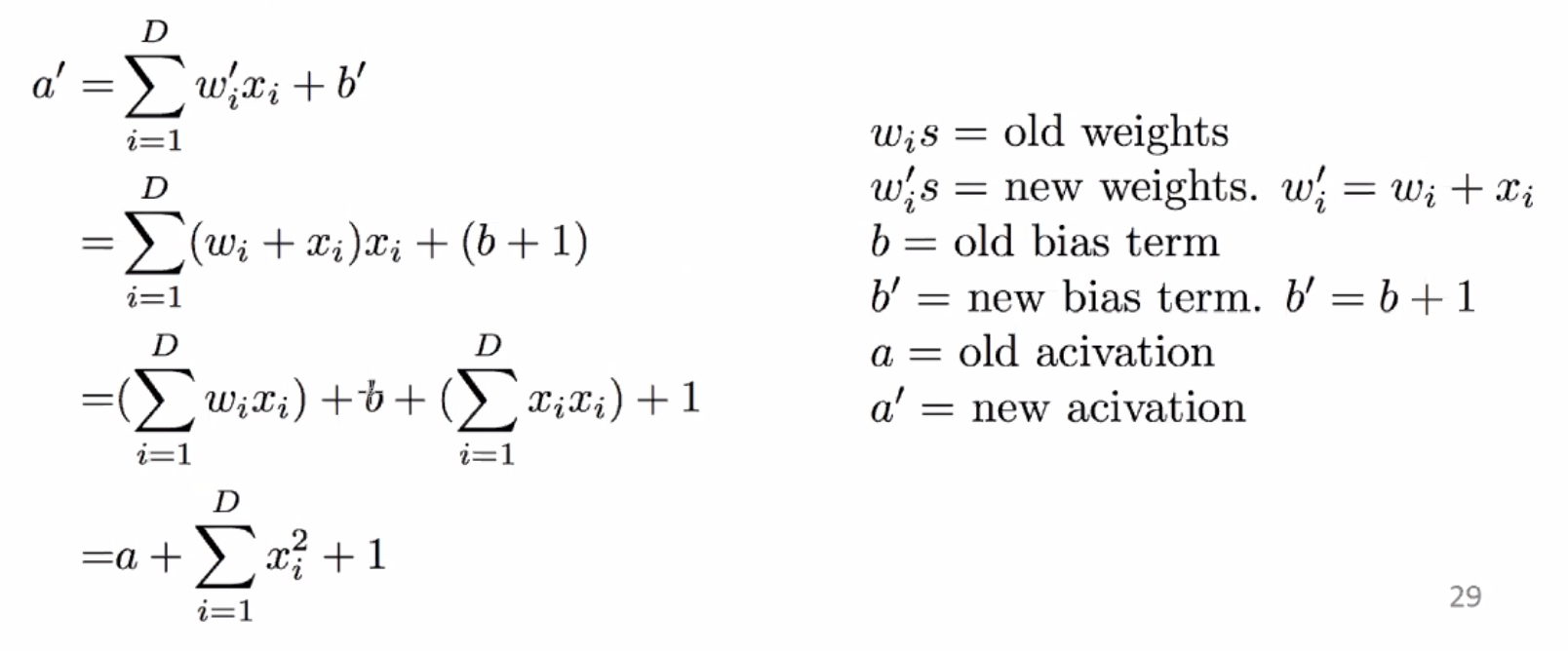So for the given example, the activation is improved by a factor of positive $$\sum_{i=1}^D x_i^2 + 1$$, bringing the prediction closer to correctiveness for that one sample.

We can also control the learning rate easily using a term $$\eta$$:

$\mathbf{w} = \mathbf{w} + y \eta \mathbf{x}$

### Caveats¶

• the order of the training instances is important!
• e.g. all positives followed by all negatives is bad
• recommended to permute the training data after each iteration

## Example¶

x1 x2 y  wx  w (after update, if any)
-------------------------------------
<0, 0>
1  3  +   0 <1, 3>
2  3  -  11 <-1, 0>
-3  1  +   3 <-1, 0>
1 -1  -  -1 <-1, 0>


## Convergence¶

We can define convergence as when going through the training data once, no updates are made

If the training data is linearly separable, perceptron will converge - if not, it will never converge.

How long perceptron takes to converge is based on how easy the dataset is - roughly, how separated from each other the two classes are (i.e. the higher the margin is, the easier the dataset is, where margin is the distance from the hyperplane to a datapoint)

### Proof¶

Overview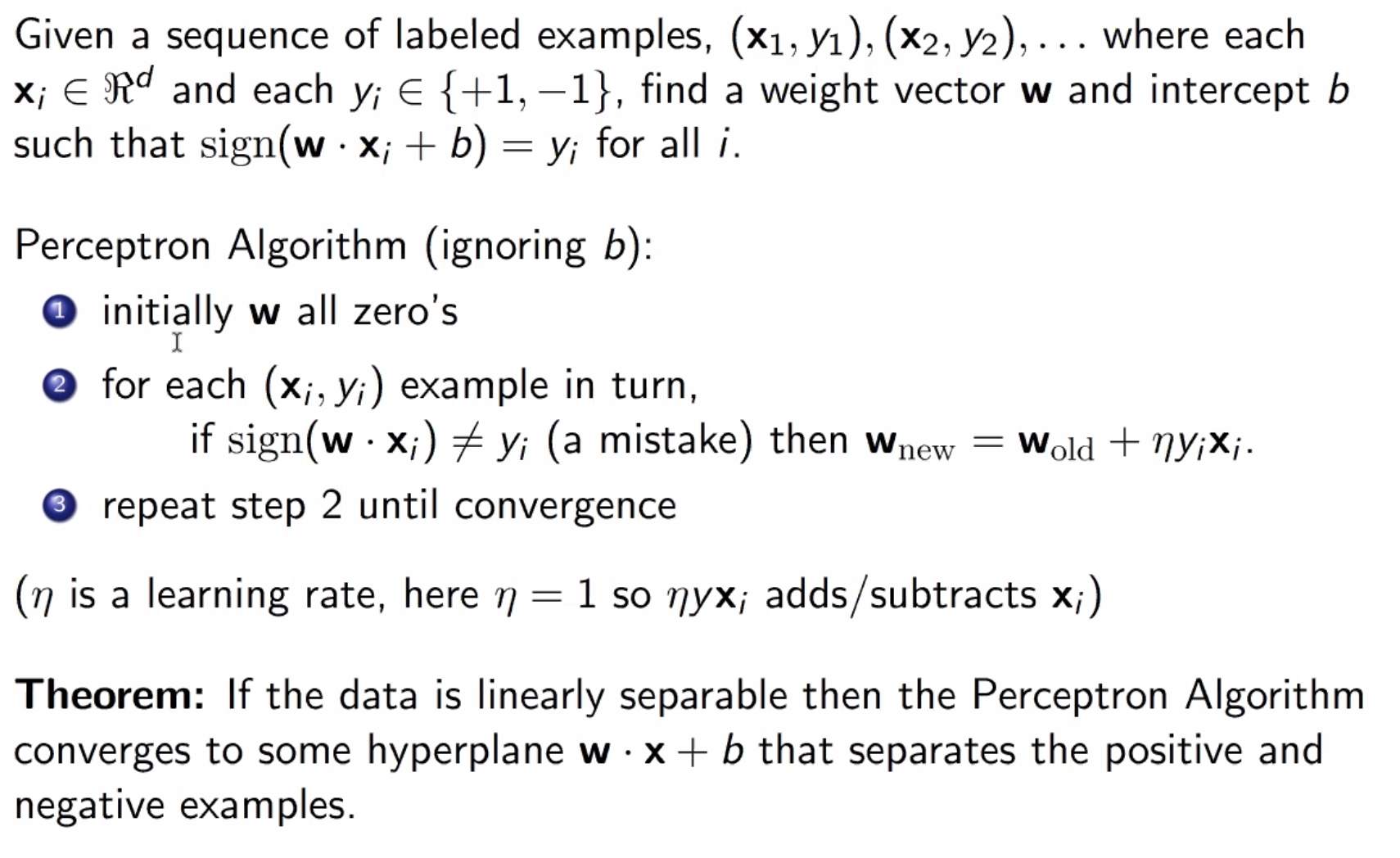Steps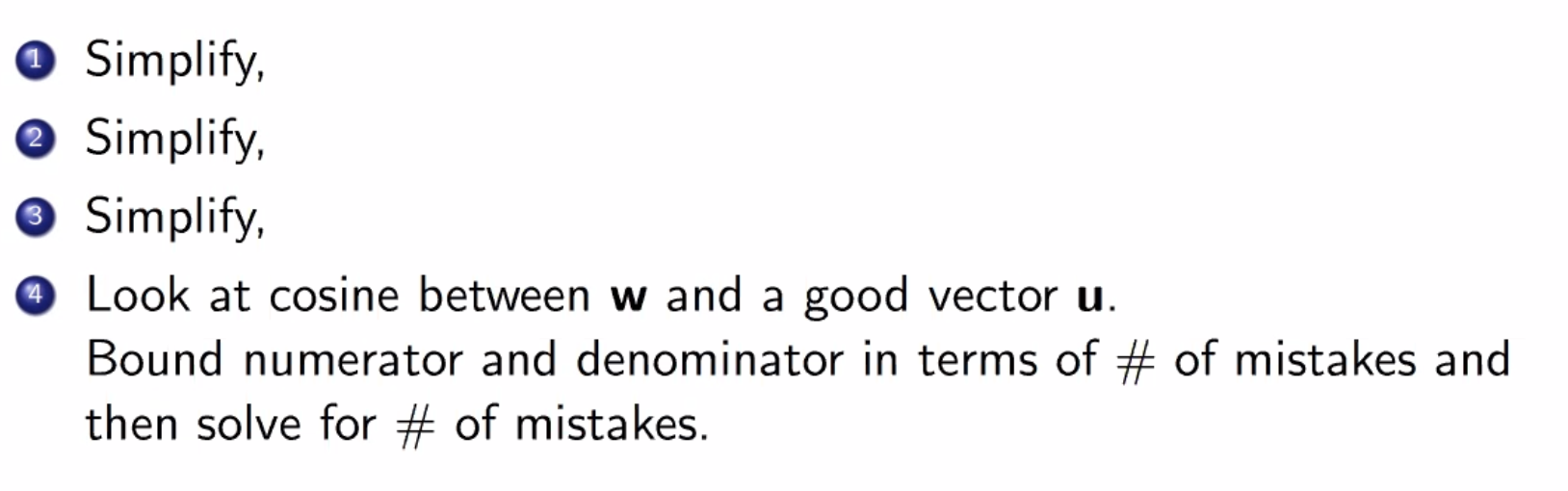Simplification 1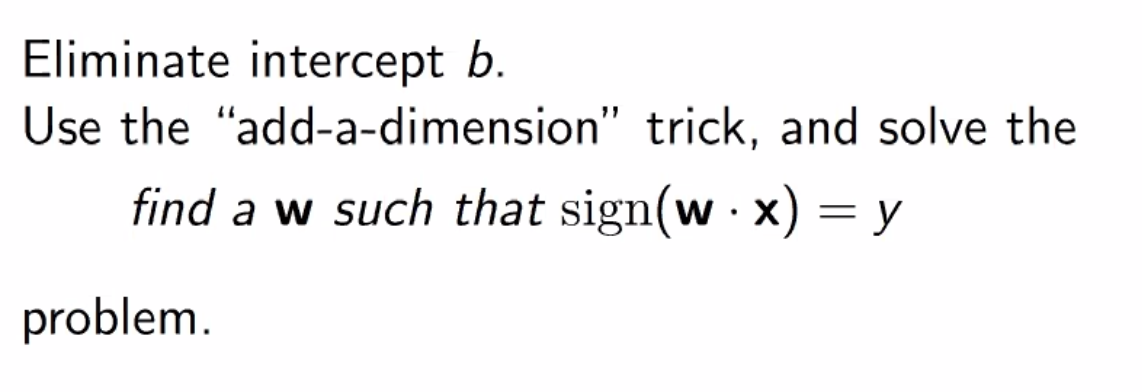Simplification 2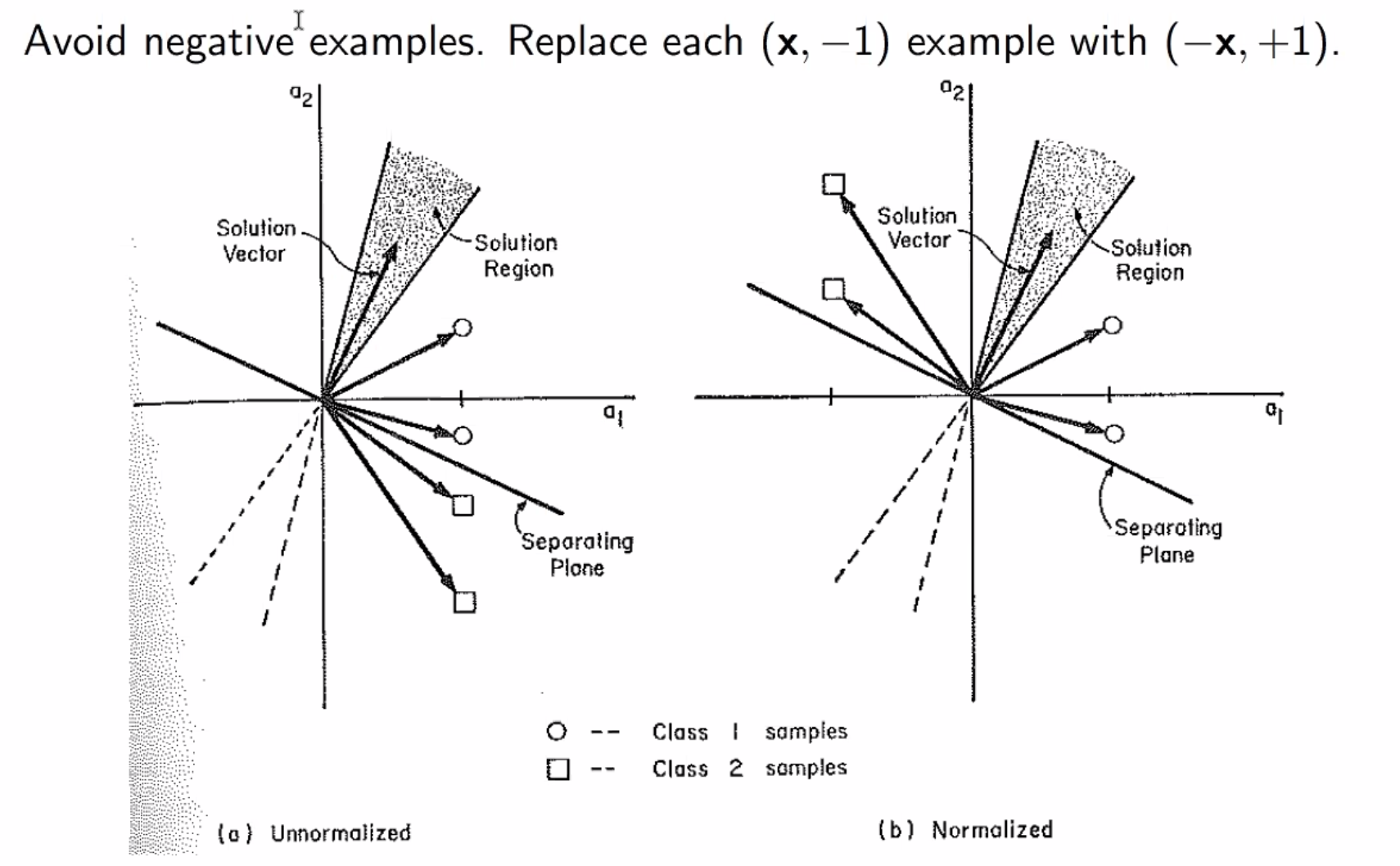Simplification 3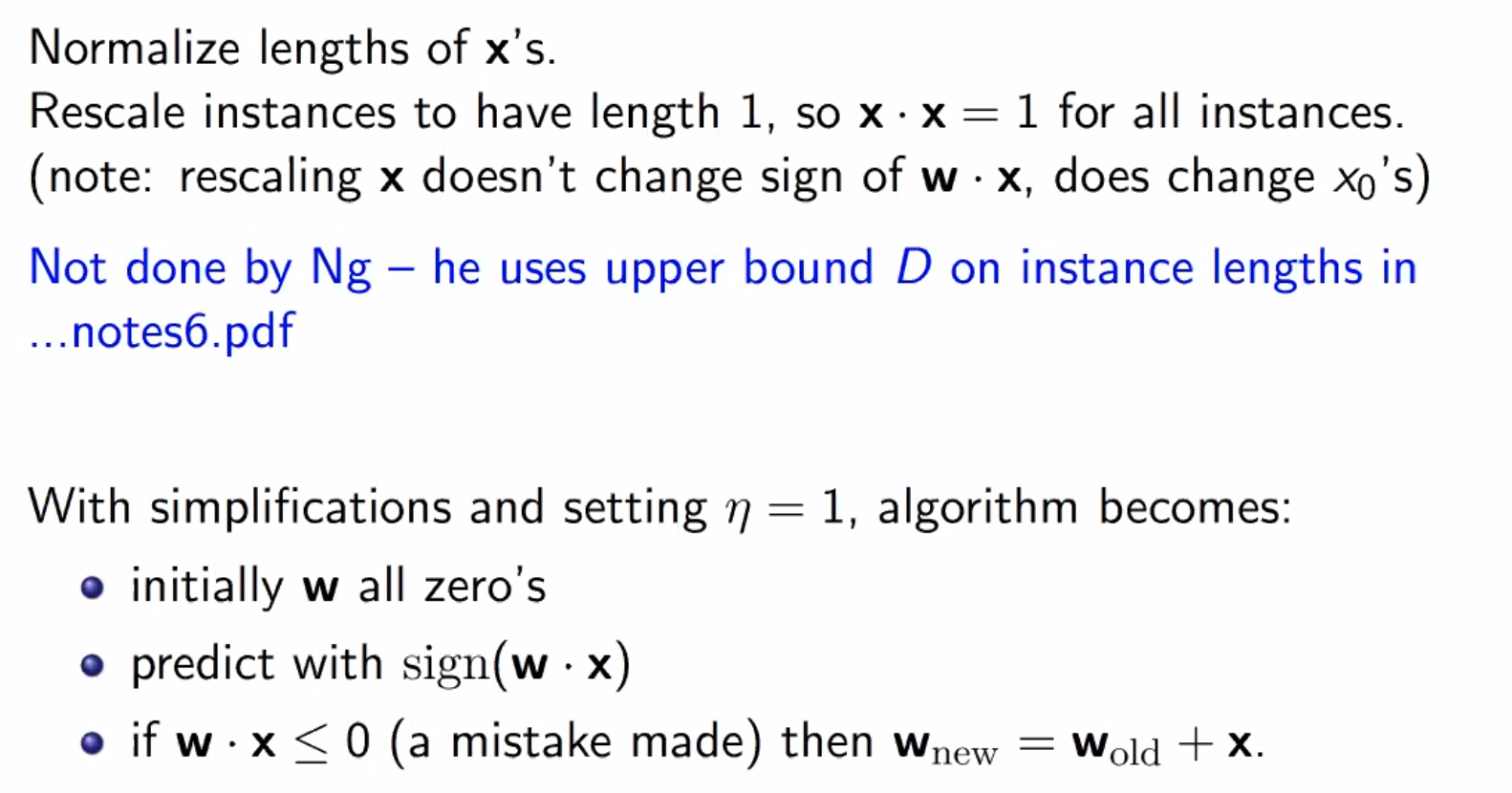Analysis Setup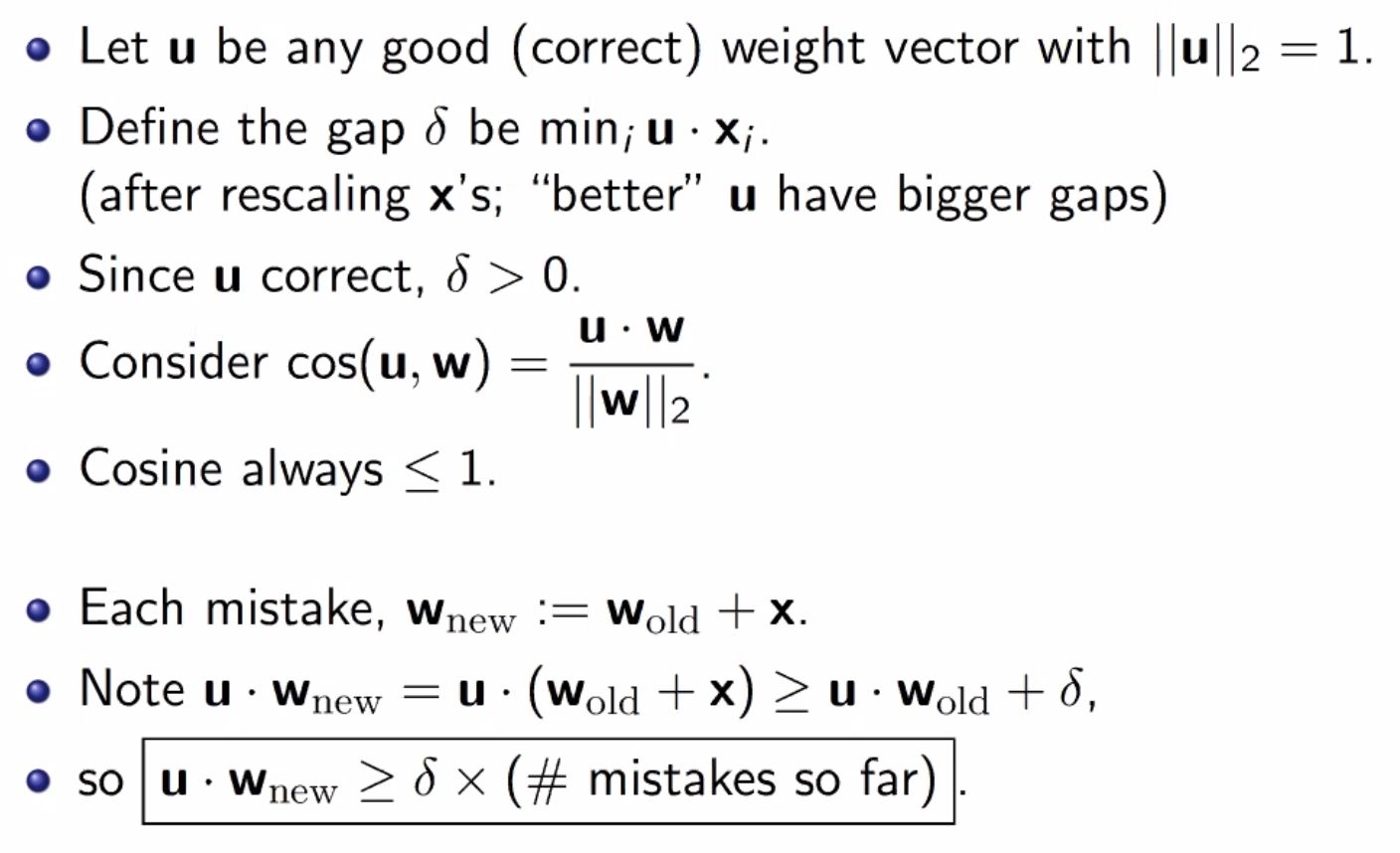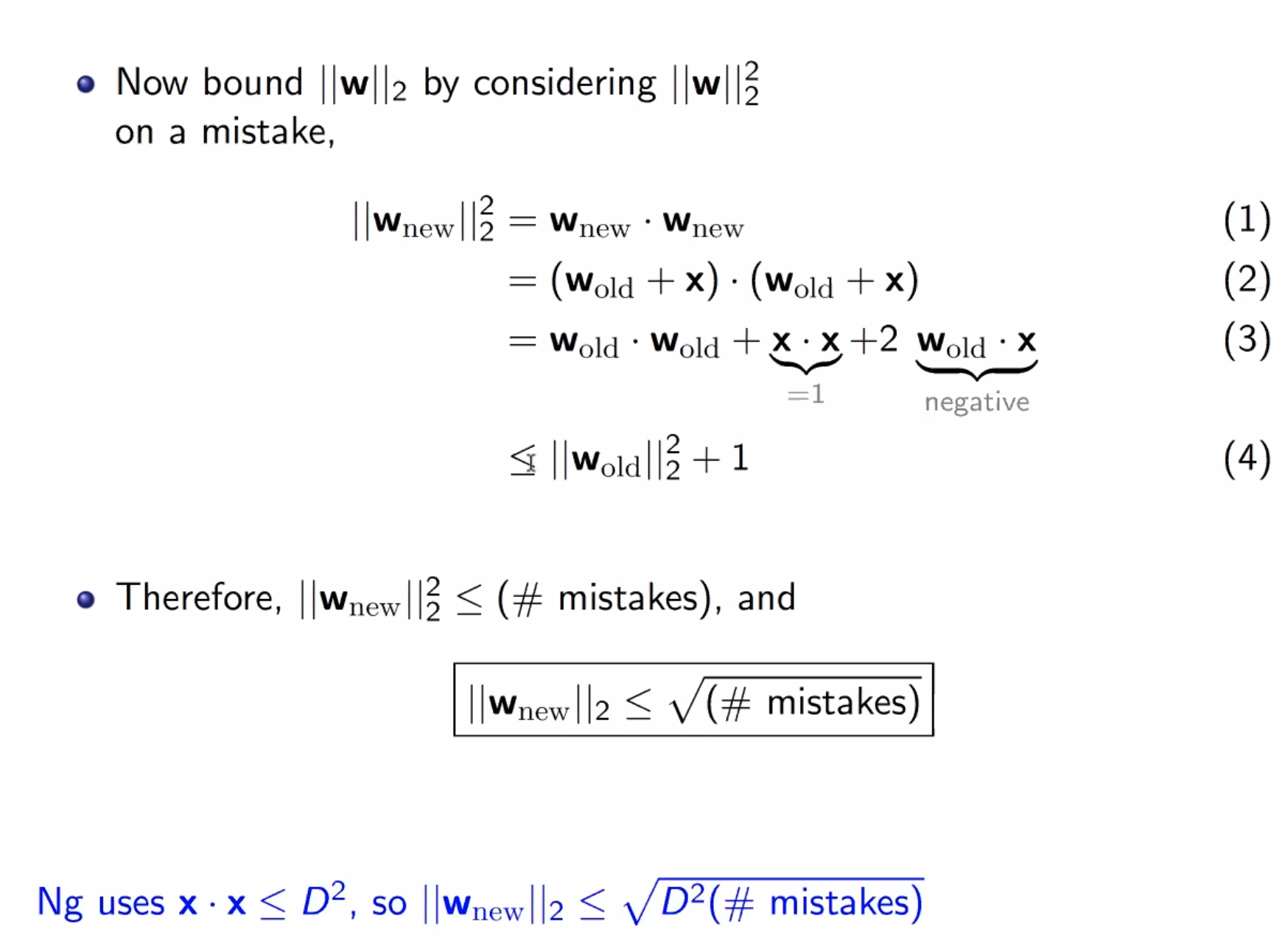Finishing Up